Previous: 7.1.2.1 Schrödinger: Transparent BCs Up: 7.1.2 Absorbing Boundary Conditions Next: 7.1.3 Comparison: Schrödinger, Riccati

### 7.1.2.2 Riccati and Prüfer: Dirichlet BCs

The boundary conditions given by 7.5 translate to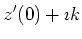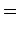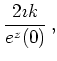(7.6)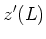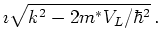(7.7)

If we set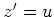we get (with constant mass in the electrodes)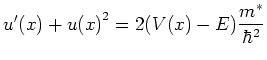(7.8)

with the Dirichlet boundary condition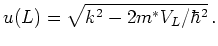(7.9)

Solving Equation 7.8 fixes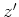. The value of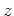is fixed up to an additive constant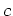, which is then determined by the second boundary condition. For the Prüfer Equation 6.39 we get the Dirichlet boundary condition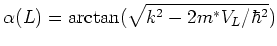(7.10)

We stress that with inflow boundary conditions the wave function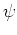cannot have nodes, hence in this case the Riccati equation has an everywhere defined solution. The main advantage with respect to the Schrödinger equation is that it is a (nonlinear) first order equation with a Dirichlet boundary condition.

We have implemented both the Prüfer and the Riccati equation as an alternative to the Schrödinger equation. The results are reviewed in the next section.